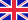•# Astrodynamics

Autor: Source: Wikipedia

Source: Wikipedia. Pages: 106. Chapters: Geosynchronous orbit, Apparent retrograde motion, Potential energy, Equinox, Geostationary orbit, Inertial frame of reference, Low Earth orbit, Escape velocity, Inclination, Statite, Apsis, Orbital elements, Orbital... Viac o knihe

Na objednávku, dodanie 2-4 týždne

23.38 €

bežná cena: 27.50 €

## O knihe

Source: Wikipedia. Pages: 106. Chapters: Geosynchronous orbit, Apparent retrograde motion, Potential energy, Equinox, Geostationary orbit, Inertial frame of reference, Low Earth orbit, Escape velocity, Inclination, Statite, Apsis, Orbital elements, Orbital period, Gravity assist, Hohmann transfer orbit, Trans Lunar Injection, Orbital mechanics, Tsiolkovsky rocket equation, List of orbits, Delta-v budget, Geocentric orbit, Space geostrategy, Gravitational potential, Orbital eccentricity, Space rendezvous, Interplanetary Transport Network, Specific orbital energy, Semi-major axis, Ground track, Elliptic orbit, Molniya orbit, Perturbation, Orbital node, Parking orbit, Orbit equation, Eccentric anomaly, Standard gravitational parameter, Geostationary transfer orbit, Sun-synchronous orbit, Beta angle, Pendulum rocket fallacy, Osculating orbit, Sunrise equation, Bi-elliptic transfer, Gravity drag, Orbital station-keeping, Longitude of the ascending node, Laplace plane, Klemperer rosette, Orbital state vectors, True anomaly, Equation of the center, Tisserand's Criterion, Lissajous orbit, Proper orbital elements, Hyperbolic trajectory, Parabolic trajectory, Vis-viva equation, Orbital inclination change, Halo orbit, Minimum orbit intersection distance, Jacobi coordinates, Circular orbit, Graveyard orbit, Polar orbit, Propellant mass fraction, Sphere of influence, Orbital maneuver, Mean anomaly, Argument of periapsis, Semi-minor axis, Tisserand's parameter, Low energy transfer, Mass ratio, Transposition, docking, and extraction, Porkchop plot, Specific relative angular momentum, Mean longitude, Nodal precession, Tidal shock, Orbital plane, Eccentricity vector, Longitude of the periapsis, Canonical units, Patched Conic Approximation, Characteristic energy, Trans-Earth injection, Areosynchronous orbit, Geostationary ring, Payload fraction, Astronomical algorithm, Plane of reference, Areostationary orbit, Apse line, Solar radiation pressure, Heliosynchronous orbit, True longitude, Non-inclined orbit, Central body, Orbiting body, Perigalacticon, Semi-synchronous orbit, Subsynchronous orbit, Orbit phasing, Near equatorial orbit, Galactocentric distance, Path-constrained rendezvous. Excerpt: In physics, an inertial frame of reference (also inertial reference frame or inertial frame or Galilean reference frame) is a frame of reference that describes time homogeneously and space homogeneously, isotropically, and in a time independent manner. All inertial frames are in a state of constant, rectilinear motion with respect to one another; they are not accelerating (in the sense of proper acceleration that would be detected by an accelerometer). Measurements in one inertial frame can be converted to measurements in another by a simple transformation (the Galilean transformation in Newtonian physics and the Lorentz transformation in special relativity). In general relativity, an inertial reference frame is only an approximation that applies in a region that is small enough for the curvature of space to be negligible. Physical laws take the same form in all inertial frames. In a non-inertial reference frame the laws of physics depend upon the acceleration of that frame of reference, and the usual physical forces must be supplemented by fictitious forces. For example, a ball dropped towards the ground does not go exactly straight down because the Earth is rotating. Someone rotating with the Earth must include the Coriolis force to predict the h...

• Vydavateľstvo: Books LLC, Reference Series
• Rok vydania: 2011
• Formát: Paperback
• Rozmer: 246 x 189 mm
• Jazyk: Anglický jazyk
• ISBN: 9781157059172

Generuje redakčný systém BUXUS CMS spoločnosti ui42.